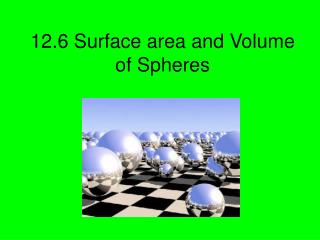DownloadDownload Presentation12.6 Surface area and Volume of Spheres

# 12.6 Surface area and Volume of Spheres

Télécharger la présentation## 12.6 Surface area and Volume of Spheres

- - - - - - - - - - - - - - - - - - - - - - - - - - - E N D - - - - - - - - - - - - - - - - - - - - - - - - - - -
##### Presentation Transcript

1. 12.6 Surface area and Volume of Spheres

2. Definitions • Sphere: is the locus pf points in space that are a given distance from a point. The center of the sphere is that point. • Radius of a sphere: is a segment from the center to a point on the sphere. • Chord of a sphere: is a segment whose endpoints are on the sphere. • Diameter: is a chord that contains the center.

3. Cont. • If a plane intersects a sphere, the intersection is either a single point or a circle. If the plane contains the center of the sphere, then the intersection is a Great Circle. • Hemisphere: two congruent halves of a sphere.

4. Theorems • 12.11: The Surface area S of a sphere with radius r is S=4πr² • 12.12: The volume V of a sphere with the radius r is V=4/3πr³

5. Examples Find the Surface Area S=4πr² S= 4(3.14)(22²) S=4(1519.76) S=6079.04inches

6. Find Volume of Sphere V=4/3πr³ V=4/3(3.14)(22³) V=4/3(33434.72) V=44579.626

7. Pg.762 and 763 #10-29 skip # 17